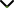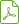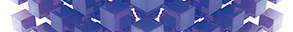# Optimization Algorithms Combining (Meta)heuristics and Mathematical Programming and Its Application in Engineering

Status
Published

Guest Editors

1Pontificia Universidad Católica de Valparaíso, Valparaíso, Chile

2Nanyang Technological University, Nanyang, Singapore

3CONICET, Buenos Aires, Argentina

# Optimization Algorithms Combining (Meta)heuristics and Mathematical Programming and Its Application in Engineering

## DescriptionComplex optimization problems can be tackled by means of mathematical programming methods as well as by means of (meta)heuristic methods. On the one hand, mathematical programming methods give us guarantee of optimality, while (meta)heuristic methods do not. On the other hand, heuristic methods can handle large and complex optimization problems, while mathematical programming methods tend to fail as the size of the optimization problem in hand increases. Thus, it makes sense to combine these two strategies to obtain better solutions to the problem that is being addressed. During the last two decades or so, algorithms that either include mathematical programming solvers into (meta)heuristic frameworks or include (meta)heuristic concepts within mathematical programming methods have demonstrated to be very effective in solving large complex optimization problems. These hybrid algorithms are also called matheuristics.

The aim of this special issue is to publish high-quality papers that combine (meta)heuristic methods and mathematical programming to solve complex optimization problems. Reviews on this topic are also welcome.

Potential topics include but are not limited to the following:

• Theoretical issues on matheuristic methods
• Novel algorithms combining mathematical programming and (meta)heuristics methods
• Mixed integer programming and (meta)heuristic methods
• Guided search and mathematical programming
• Mathematical programming solvers embedded into (meta)heuristic frameworks
• Hybrid algorithms for big data
• Hybrid algorithms for deep learning
• Hybrid algorithms applied to real problems from industryDownload Special IssueJournal metrics
Acceptance rate27%
Submission to final decision64 days
Acceptance to publication34 days
CiteScore1.800
Journal Citation Indicator0.400
Impact Factor1.305Author guidelinesEditorial boardDatabases and indexing

Article of the Year Award: Outstanding research contributions of 2020, as selected by our Chief Editors. Read the winning articles.